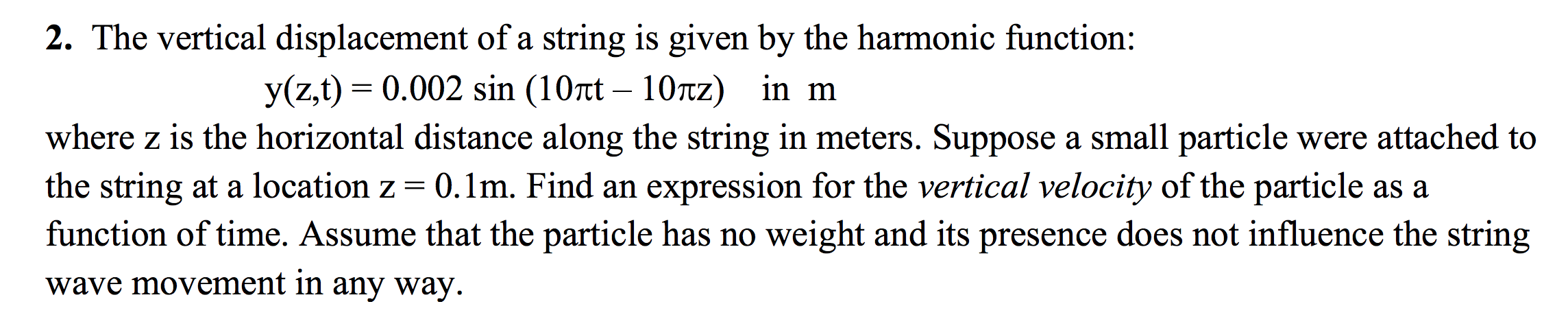Home / Answered Questions / Other / 2-the-vertical-displacement-of-a-string-is-given-by-the-harmonic-function-y-z-t-0-002-sin-10rt-10tz--aw614

# (Solved): 2. The Vertical Displacement Of A String Is Given By The Harmonic Function: Y(z,t) = 0.002 Sin (10rt...2. The vertical displacement of a string is given by the harmonic function: y(z,t) = 0.002 sin (10rt â€“ 10tz) in m where z is the horizontal distance along the string in meters. Suppose a small particle were attached to the string at a location z=0.1m. Find an expression for the vertical velocity of the particle as a function of time. Assume that the particle has no weight and its presence does not influence the string wave movement in any way.

We have an Answer from Expert